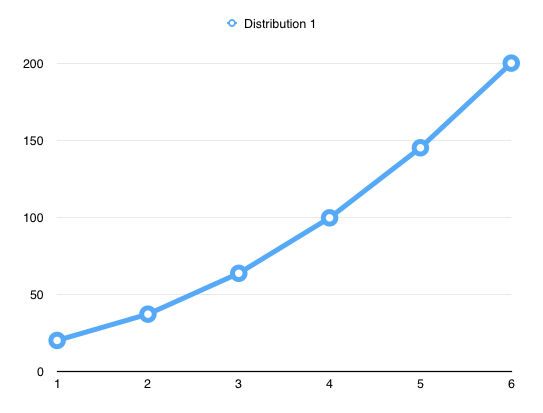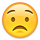#### Howdy, Stranger!

It looks like you're new here. Sign in or register to get started.

# A new stem weight distribution?

edited September 2016
Hello all,

I just had a look at @PabloImpallari's stem weight calculator, and noticed something odd (I think).

So far, I've got a regular and an extrabold, but the only fields available are 'thin' and 'black', so I just use those instead.
Thin 92, Black 162, steps: 4     ->     92, 113, 137, 162  (Impallari Formula)

If I then pretend that I have a light weight at 42, and increase the steps to 7, this happens
Thin: 42, Black 162, steps 7     ->     42, 54, 71, 92, 116, 140, 162

So, in the second configuration, focussing on the last four values, the steps are different than in the first configuration (even though the regular is still at 92). Logically, I don't think this should be the case. The same problem would occur with the De Groot method. I may be wrong, though, so feel free to correct me.

If I am right, however, a better formula for calculating stem weights would be useful. I have used the following distribution for Proza and Proza Display, but only came up with the formula just now. Maybe the whole thing is nothing new, I don't know.

Assume that a light weight is 40, and a black weight is 200 (difference is thus 160), and there are 4 steps (in Pablo's calculator, this would be considered 5 steps. I say there are 5 values, thus 4 steps from value to value).

A linear approach looks like this:
1=40      2=80      3=120      4=160      5=200
+40         +40          +40          +40

My approach looks something like this:
1=40      2=80      3=125      4=175      5=230
+40        +45          +50          +55
Then, to make sure we stay within the 40 to 200 bounds, I multiply each of the figures in the line directly above this, by (160/190=0.84), (that's the original difference divided by the 'new' difference) which gives:
+34        +38          +42         +46
Thus, the instances become:
1=40      2=74      3=112      4=154      5=200

So far for the basic explanation. Now, to get to these instances right away, I created a formula. In the formula '#' means the number that is before the = sign. X is a value that can be freely chosen (in the example above it was 5). Y is the value of the previous (lighter) instance. Here goes:

1=40

2=Y+(difference/steps+X*(#-2))  *     (difference/((steps-1)*steps)/2*X+difference)
40 +   (          40        +5 *  0   )   *    (    160     /                      190                       ))    =  74

3=Y+(difference/steps+X*(#-2))  *     (difference/((steps-1)*steps)/2*X+difference)
74 +   (          40        +5 *  1   )   *    (    160     /                      190                       ))    =  112

4=same formula

5=same formula

Now, if wanted to add an even lighter weight, it would be set at 10, and X would still be 5, and all the other instances would remain the same. Even if I wanted to add a number of darker or lighter weights than I already have, the 'original' instances would remain the same. To illustrate:
1=10
2=10 + (190/5+0)*(190/240)=40
3=40 + 190/5+5)*(190/240)=74
etc.

I think this distribution has two advantages:
- It provides a flexible X, which determines how much you want to deviate from the linear distribution. The X can be varied for each typefamily.
- Adding lighter or darker weights does not change the distribution of the existing weights.
- A tool to do this automatically would be helpful, at least for me.

I hope my calculations and assumptions are all correct. If not, please explain in simple language. I'm somewhat brainfried. Sorry for the long post, but I did my best to explain it clearly.

Cheers!

• edited September 2016
edited original post
• For lack of a better tool, here's an excel sheet (generated from Apple's 'Numbers'), that maybe makes it easier to use/understand.
• Are you saying instead of a constant ratio, you prefer a ratio where the difference between steps increases by an arithmetic amount? Is that amount a constant or a second ratio in itself?
• That amount is a constant, making the diagram look something like this the image attached (X=20).• The problem I have with a constant is that the desired degree of stem variation at thin weights is different than at heavy weight sizes.  I don't see how a constant can be correct.  It may make your sample sheets look nice though, if that is what you want.
• Jasper de Waard:
The same problem would occur with the De Groot method.

This problem does not occur with the De Groot method. Example:

Thin 92, Black 162, steps: 4     ->     92, 111, 134, 162    (Lucas De Groot Formula)

Thin: 52, Black 162, steps 7     ->     52, 63, 76, 92, 111, 134, 162    (Lucas De Groot Formula)

• I tried Pablo's tool and I didn't like much the results that I got.
• Pablo's tool is just that, a tool, not a panacea. I can see his and Lucas's thinking and appreciate them both for their efforts and generosity in making their work available to us without charge.  As usual, we are all expected to take it from there and make our own design decisions.
• edited September 2016
The other issue today is stem widths that translate for web use. We make an effort to find stem width to sizes that grid-fit at 1px, 2 px, 4px, 8px, etc., so that we get the best web output.  This may not coincide with a smooth progression of weights for printing specimen sheets.
• edited September 2016
Hi @Jasper de Waard
Now I'm back at home and had a little more time to fully read your initial post.

I've experimented with a similar formula as yours in my initial attempts when creating the calculator. Basically your formula is a "softer" version of the Lucas formula. By "softer" I mean this:This links goes to a early beta version of my calculator:
http://www.impallari.com/familysteps/index-v2.php
If you input the same values as in your example (40 to 200 in 5 steps) you will see that in the 25% column you get the same results (40, 75, 112, 154, 200 - In step 2: 74 to 75 may be a rounding difference).

All this area is explorable, and I happy to see people exploring it!You can also make the opposite of a "softer" formula, and go deeper in the belly of the curve, and have an even bigger explorable area.

The reason I went for a Ogee curve instead of a constant, was perfectly stated by @Chris Lozos in his comment. That's also the same reason why the Lucas formula (and yours) is extendable (allowing to add more styles before and after the extremes) and mine is not (requiring people to plan in advance).
• @Ben Blom Thanks for correcting me!

@PabloImpallari Ah, that beta link is interesting! I've been trying to figure out a way to have a curve that looks like the De Groot curve (or your curve, which is of course related), where the 'curviness' is flexible, and the results are 'extendable', which has to be possible. Not much luck so far, though. My math skills are letting me downOn a more general note:

It's an interesting 'debate' between keeping the differences between weights optically the same, thus creating nice spreadsheets and such, and just picking a set of instances by hand/eye.

The first does maybe seem a little too much involved with marketing, and not enough with actual functionality, but I like to be able to use my SemiBold next to a Light, in the same way that I would use a Bold next to a Regular, or a Black next to a Bold, etc. Having an optically 'smooth' scale of weights does ensure that. An important condition, though, is that the Bold looks perfect next to the Regular to begin with.

Also, I wonder how you pick your weights by eye? How do you know that a certain instance is the 'right' instance for a weight (apart from the obvious regular/bold combination)?
•  I like to be able to use my SemiBold next to a Light, in the same way that I would use a Bold next to a Regular
That is what only Lucas’ formula will give you.

• Should this be added to Insert Instances as ‘Jasper softening’, a shift towards the linear distribution?
• @Georg Seifert: This is what Lucas' formula does in theory, but the curve that results from his formula is a fixed one, whereas I believe that the amount of curvature needs to be different for different typefaces. I'm sure there is a way to make that work mathematically, but I haven't been able to find an elegant solution so far. (Pablo's method of simply interpolating between the linear and the curved line seems logical to me, but I don't understand why it is not 'extendable'.

@Rainer Erich Scheichelbauer That seems too much of an honor for something that basically Pablo has already done here: http://www.impallari.com/familysteps/index-v2.php If this would be included in Glyphs, I would like to be able to just type in a percentage (or some other value), though• edited September 2016
Also, I wonder how you pick your weights by eye? How do you know that a certain instance is the 'right' instance for a weight
That's the key question. And you don't know the answer until you test and compare
• Reading is a visual process.  It stands to reason that a visual process be used to determine the attributes of the type being read. The "how" part involves a great deal of work to gain the experience needed to make good decisions. As much as we want all the answers in a nice tidy list, reading is not tidy.
• whereas I believe that the amount of curvature needs to be different for different typefaces.
It calculates positions for each instance that the relative change in stem width is equal for each step. There is no design dependency.
If you want equal proportions, stick with Lucas, if not do something else.